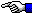Some Investigations into:

# Mathematics

math•e•mat•ics (mathe·mat´iks) — the systematic study of forms, figures, and quantities; the properties, measurement, and the relationships between them, expressed in both numbers and symbols. A collective word for all the procedures and operations of related "branches" which have historically arisen from mathematics; such as, Arithmetic, Geometry, Algebra, Trigonometry, Calculus (Differential and Integral), Set Theory, Boolean Logic, Statistics, and Topology. Often used to describe or speak of the mathematical aspects of various sciences, such as "the mathematics of physics."
[
1350-1400; MiddleEnglish mathematic from the Latin mathematica from the Greek mathematikč (téchne) scientific (craft), equivalent to mathemat- (the singular of máthema) science or knowledge + -ike, fem. of -ikos -ic ].

[Composite definition by The Starman; based on many different sources.]

### "The universe is a book open for us to gaze upon, but it cannot be understood unless one first learns its language, the language of Mathematics; written in numbers and geometric figures, without which it is impossible to understand a single word of it. "

—— Our paraphrase of Galileo Galilei in Il Saggiatore (1623)

OK, pull out some paper, pencils, and propositions (?)
I mean, get ready for a few interesting topics in the history of this "language!"

## Mathematics for Basic Electronics

New project; only basic formulas and examples for simple DC circuits at this time.
But you'll find notes on how current, voltage, resistance and power are defined under the SI system of Physics.

The Pi (π) Files

# Exact and verifiable values of Pi, Pi Programs and an essay on Pi ( Also includes Facts and Figures about Pi )

"Recreational Math?"

 “Anyone who regards games simply as games and takes work too seriously has grasped little of either.” So wrote the German poet Heinrich Heine over a century ago. In today's world the division of work and play persists. Old prejudice still holds that the playing of games is an activity for children, not useless perhaps, but certainly not the responsible and serious work of adults. Heine intuitively foresaw what [psychologists today have] since asserted. Games are not only necessary for the development of a child's self-awareness; they are also needed by adults, especially when their work is repetitive and uncreative. ...Those games based on mathematical or logical principles... are among the most absorbing and creative.    Indeed, the great mathematicians and scholars of the past often applied their skills to the solution of logical and mathematical games. [ I've lost the reference for who said this! Any ideas? ]

You Have All the Money You'll Ever Need!
Learn about an important Mathematical Rule.

Repeating Decimal Fractions Includes Chart of All Fractions From 1/2 through 1/66.

Truthteller, Liar and Randomizer This problem actually has more to do with Logic than Math, but Mathematics does require you to think logically, so... have a try at this.

How many Squares can you find in this Diagram?

Four 4s These problems actually use Logic more than Math, but Mathematics is required to both arrive at and verify the answers.Back to The Starman's Realm

Remember, you can always write to me at my Feedback page here !